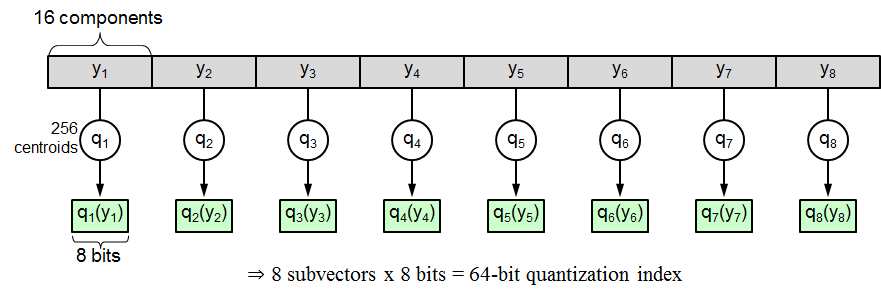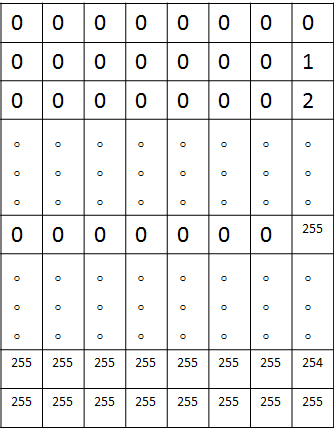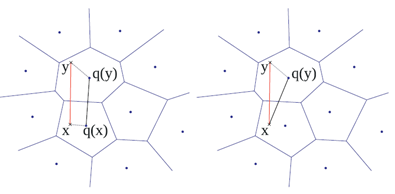## 1. 空间切分int ds= d / nsq;
for ( int i = 0; i < nsq; i ++ )
{
for ( int j = 0; j < ds; j ++ )
{
vs.push_back( vtrain.row( i*ds + j ) );
}
}
//vtrain的转置是原始128维向量，vs是其中的一个子向量

## 2. 量化

kmeans( vs, ks, labelssub,
TermCriteria( CV_TERMCRIT_EPS+CV_TERMCRIT_ITER, 50, 0.001 ),
3, KMEANS_PP_CENTERS, centers );

float my_norm_L2( Mat mat1, Mat mat2 )
{
int d = mat1.cols;
float sum_d = 0.0;
for ( int i = 0; i < d; i ++ )
{
float pp = mat1.at<float>( 0, i ) - mat2.at<float>( 0, i );
sum_d = sum_d + pp*pp;
}
return sum_d;
}

## 3. 压缩## 4. 距离计算Mat sumidxtab( Mat &D, Mat&x, Mat & dis )
{
dis = Mat::zeros( 1, x.rows, CV_32FC1 );
for ( int i = 0; i < x.rows; i ++ )
{
float distmp = 0;
for ( int j = 0; j < D.cols; j ++ )
{
distmp = distmp + D.at<float>(  x.at<int>( i, j ), j ) ;//ADC距离计算方式；
}
dis.at<float>( 0, i ) = distmp;
}
return dis;
}
//D为查找表，x为压缩向量，dis为最终的距离  

## 5. 总结

Last modification：March 17th, 2021 at 01:42 pm